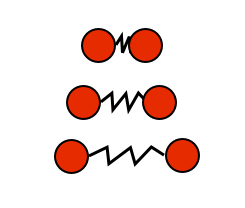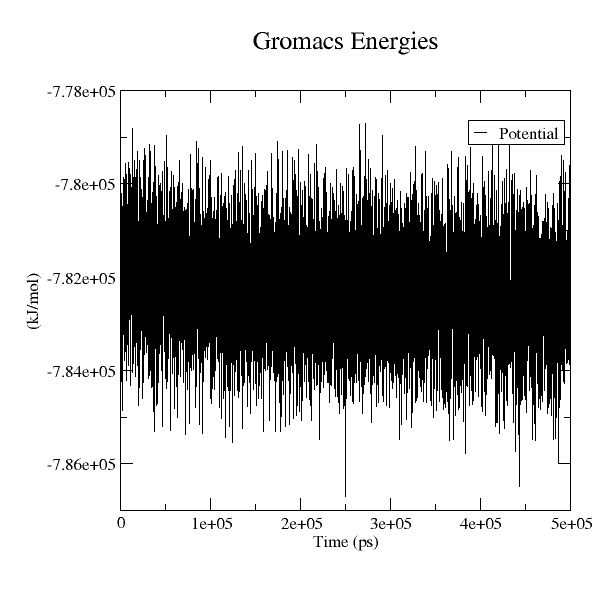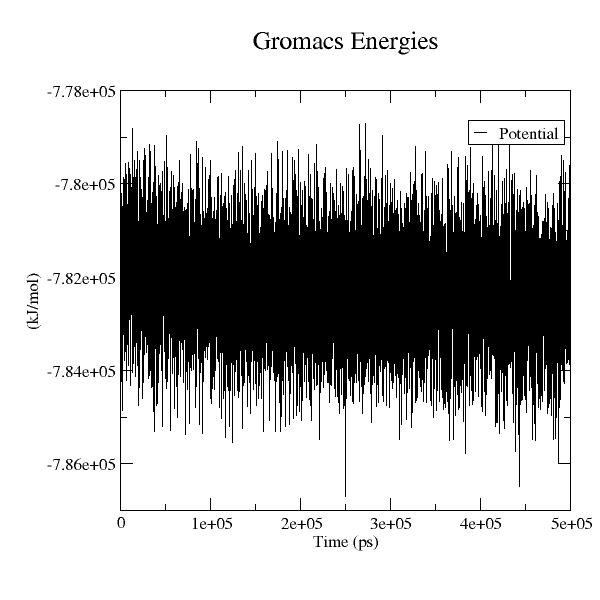# 8.Molecular Dynamics Simulations¶

## Introduction¶

Consider two small masses, connected by a spring as shown here:The mass on the left is $i$, and on the right is $j$.

The potential energy of the spring holding them together is given by $$U(x_{ij})=\frac{1}{2}k_{ij}\left(x_{ij}-b_{ij}\right)^2,$$ where $$r_{ij}=x_i-x_j,$$ and $k_{ij}$ and $b_{ij}$ are constants unique to the type of spring that connects masses $i$ and $j$.

This equation is often used as an approximation for the potenetial energy of a covalent bond between two atoms. Let $i$ be a carbon atom, and $j$ be an oxygen atom. On average, these atoms are 0.122 nm apart from each other, and the spring constant is 570 kcal/mol nm$^2$. If $i$ and $j$ were both carbons, they would be 0.152 nm apart at equilibrium, with a spring constant of about 317 kcal/mol nm$^2$.

## Exercise 1¶

Make a plot of the potential energy of both a carbon-carbon and a carbon oxygen bond on the same plot, with axes with properly labeled in the correct units.

In :
%plot --format svg -w 600 -h 400

In :
r_0=0.122;  % in nm
k=570;      % in kcal/mol nm^2
r=[r_0-0.005:0.0001:r_0+0.005];
plot(r,0.5*k*(r-r_0).^2,'b-');
xlabel("r_{ij}, nm");
ylabel("U, kcal/mol");
legend("carbon-oxygen");
pbaspect([1 0.75 1]);

In :
%plot --format SVG -w 800 -h 300
subplot(1,2,1);
r_0=0.122;  % in nm
k=570;      % in kcal/mol nm^2
r=[r_0-0.005:0.0001:r_0+0.005];
plot(r,0.5*k*(r-r_0).^2,"b-;carbon-oxygen;");
xlabel("r_{ij}, nm"); ylabel("U, kcal/mol"); pbaspect([1 0.75 1]);

subplot(1,2,2);
r_0=0.152;  % in nm
k=317;      % in kcal/mol nm^2
r=[r_0-0.005:0.0001:r_0+0.005];
plot(r,0.5*k*(r-r_0).^2,"r-;carbon-carbon;");
xlabel("r_{ij}, nm"); ylabel("U, kcal/mol"); pbaspect([1 0.75 1]);


## Exercise 2¶

At what distace apart from each other $r_{ij}$ is the minimum of $U(r_{ij})$ for both of these cases?
First, show the steps to solve it analytically here, then solve it using octave's fsolve function.

$$\frac{d U}{d r_{ij}} = k_{ij}\left( r_{ij}-b_{ij} \right) = 0 \quad \leadsto \quad r_{ij}=b_{ij}$$

In :
% For carbon-oxygen bond
function f = CO(x)
k=570;
b=0.122;
f=k*(x-b);
end

[x, fval] = fsolve (@CO, 0.1);
x

x =  0.12200

In :
% For carbon-carbon bond
function f = CC(x)
k=317;
b=0.152;
f=k*(x-b);
end

[x, fval] = fsolve (@CC, 0.1);
x

x =  0.15200


Consider: What does the value fval represent? What should it be exactly? Why isn't it the value you would expect?

## Ar-Ar interaction potential¶

Now consider that atoms $i$ and $j$ are both the element argon. Being noble gases, they cannot form a covalent bond. But quanutm mechanics says they do interact with each other. We can use the Lennard-Jones potential, a mathematically simple model that approximates the interaction between a pair of neutral atoms: $$U(x_{ij})=\frac{a_{ij}}{x_{ij}^{12}}-\frac{b_{ij}}{x_{ij}^{6}}$$ For two argon atoms, typical vaues would be $a=2.266\times10^{-6}$ kcal nm$^{12}$/mol, and $b=1.467\times10^{-3}$ kcal nm$^{6}$/mol

## Exercise 3¶

Make a properly labeled plot for this potential, showing clearly the region where $U(r_{ij})$ drops below 0.

In :
a=2.266e-6;
b=1.467e-3;
% V=0, or x0^6=a/b or
x0=(a/b)^(1/6);

x=linspace(0.95*x0,3*x0,1000);
U=a*x.^(-12)-b*x.^(-6);
[Um,i] = min (U);
xm = x(i);

hold on;
plot(x,U,"b-;Lennard-Jones potential;");
plot([x0;3*x0],[0;0],sprintf("r:;U(r_{ij}) < 0 for r_{ij}>%.3f;",x0));
plot([xm;xm],[0;Um],sprintf("r-;minimum at r_{0}=%.3f;",xm));
hold off;

xlabel("r_{ij},  nm"); ylabel("U(r_{ij}),  kcal/mol");


At what distance apart from each other $x_{ij}$ is the minimum of $U(x_{ij})$ for argon? First, show the steps to solve it analytically here, then solve it using octave's fsolve function.

Analytically, $$\frac{dU}{dx} = -12 \frac{a_{ij}}{x_{ij}^{13}}+6\frac{b_{ij}}{x_{ij}^{7}} = 0$$ and solving for $x_{ij}$ yields: $$x_{ij} = \left( \frac{2a}{b} \right)^{1/6}$$

In :
global a=2.266e-6;
global b=1.467e-3;
% V=0, or x0^6=a/b or
x0=(a/b)^(1/6);

display(sprintf("analytically, x_{ij} = %.9f",(2*a/b)^(1/6)))

function f=dAA(x)
global a b;
f=-12*a*x.^(-13)+6*b*x.^(-7);
end

[x, fval] = fsolve (@dAA, x0);

display(sprintf("numerically,  x_{ij} = %.9f",x))

analytically, x_{ij} = 0.381630695
numerically,  x_{ij} = 0.381630692

In :
global a=2.266e-6;
global b=1.467e-3;
% V=0, or x0^6=a/b or
x0=(a/b)^(1/6);

function f=AA(x)
global a b;
f=a*x.^(-12)-b*x.^(-6);
end

for N=[10 100 1000];
x=linspace(0.95*x0,3*x0,N);
U=AA(x);

% poor: this is just the nearest value in x,V to the true min(AA(x))
[Umm,i] = min (U);
xmm = x(i);

display(sprintf(" for x-grid of %4d points: U=%f @ x=%f",N,Umm,xmm))
end

% good: the precision of this does not depend on the grid in x
[xm Um]=fminsearch(@AA,x0);
display(sprintf("              exact values: U=%f @ x=%f",Um,xm))

hold on;
plot(x,U,"b-;Lennard-Jones potential;");
plot([x0;3*x0],[0;0],sprintf("r:;U(r_{ij}) < 0 for r_{ij}>%.3f;",x0));
plot([xm;xm],[0;Um],sprintf("r-;minimum at r_{0}=%.4f;",xm));
hold off;
xlabel("r_{ij},  nm"); ylabel("U(r_{ij}),  kcal/mol");

 for x-grid of   10 points: U=-0.222509 @ x=0.400438
for x-grid of  100 points: U=-0.237105 @ x=0.379317
for x-grid of 1000 points: U=-0.237433 @ x=0.381600
exact values: U=-0.237433 @ x=0.381620


## Exercise 4¶

Consider the carbon-carbon bond from above. If you fix one atom at the origin, and let the other atom move, of course it moves by simple harmonic motion of the spring. The equation of motion is given by the differential equation $$m\frac{\partial^2 r}{\partial t^2} = -\frac{\partial U}{\partial r}.$$

For a spring system, this simplifies to $$ma=-k(r-r_0)$$ where I have dropped the subscript $ij$, and where $a={\partial^2 r}/{\partial t^2}$ is the (radial) acceleration along the C-C bond.

The solution to this equation is of course the position of the atom as a function of time; $$x(t)=A\sin(\omega t)$$ where $\omega=\sqrt{k/m}$ and the amplitude $A$ is determined from the initial conditions, $r(t=0)=r_0$, and ${\partial r}/{\partial t}(0) = A\omega=v_0$, or $A=v_0/\omega$.

Can we solve the differential equation numerically? The code below solves for $x(t)$ using the Velocity Verlet method of solving second order differential equations of this type. (Pseudo code from here:https://en.wikipedia.org/wiki/Verlet_integration#Velocity_Verlet)

After giving the atom an initial position and velocity, inside the loop the position and velocity is updated many steps. The variable h is the timestep $\Delta t$.

Run the program. What happens if you increase k by a factor of 10? What happens if you increase v1 by a factor of 10 instead? Why?

What should you change if you put the actual values of $k$ and $b$ from above?</font>

In :
clear;

%Global parameters here
k=1.0;     %Spring constant (kcal/mol)
m=1.0;     %Mass (kg)
r_0=1.0;   %Equilibrium distance (nm)
v_0=0.1;   %Initial velocity (nm/s)
t_max=10;  %total observation time
N=1000;    %number of steps in the calculation

dt=t_max/(N-1);    % Timestep (s)
t=[0:dt:t_max];    % time array, from 0 to t_max in N steps
w=sqrt(k/m);       % Frequency
A=v_0/w;           % Amplitude

% istep=1:
r(1) = r1 = r_0;    %Initial position and velocity: r_0, v_0
v(1) = v1 = v_0;

km = -k/m; %Pre-calculate this outside the main loop

% iterate istep from 2 through N
for istep=2:N
% Verlet step
a1 = (km)*(r1-r_0);
r2 = r1 + v1*dt + 0.5*a1*dt*dt;
a2 = (km)*(r2-r_0);
v2 = v1 + (dt/2)*(a1+a2);

%Store new values
r(istep) = r2;
v(istep) = v2;

%Reset old values
r1 = r2;
v1 = v2;
end

% scale up the error to be visible, make a note of the scaling factor required
scale=A/max( r - (r_0+A*sin(w*t)) );

hold on;
plot(t,r,"r--;Verlet;");
plot(t,A*sin(w*t)+r_0,"b:;exact;");
plot(t,scale*(r-A*sin(w*t)-r_0)+r_0,sprintf("-g;difference x %.0f;",scale));
hold off;


## Exercise 5¶

Make a copy of the Verlet algorithm code, and modify it to find the motion of the second argon atom trapped in the Lennard Jones potential well of the first, using made up values around $b\approx20\cdot a$. You may need to plot the potential function to make sure the well is deep enough by adjusting $a$ and $b$.

You will want to start the atom at the minimimum energy position, and start it with a small velocity. Compare the initial kinetic energy of the particle with the depth of the well.</font>

## Exercise 6: Scaling up to many particles¶

Now we want to scale up these lessons. Classical molecular dynamics simulations use Newton's equations of motion to calculate trajectories of particles, starting from a defined configuration. For each particle in the system, the total force acting on it is calculated from the interactions with other particles, as described by the force field. The force divided by the mass of the particle gives the acceleration, which, together with the prior position and velocity, determines what the new position will be after a small time step. The high spatial and temporal resolution make molecular dynamics simulations useful for testing models based on experimental data, for understanding principles underlying the function and to formulate new hypotheses. Unfortunately, system sizes are limited, as are time scales.

Atomic bonds as springs and Lennard Jones interactions are just parts of a realistic force field. (See https://en.wikipedia.org/wiki/AMBER for example.) When you have 10,000 or more atoms, we need larger software packages to accomplish this task.

Follow the tutorial here: http://www.bevanlab.biochem.vt.edu/Pages/Personal/justin/gmx-tutorials/lysozyme/index.html This example will guide a new user through the process of setting up a simulation system containing a protein in a box of water, with ions. Each step will contain an explanation of input and output, using typical settings for general use.

For bonus marks, follow the tutorial, but instead of using using lysozyme, use one of the following prioin proteins; 1qlz, 1xyw, 1u3m, or 1xu0. If you do, just be sure to add the option -ignh to the pdb2gmx command.

For your protein, write down the animal species it comes from: species name

Include an image of the protein here:In "Step One: Prepare the Topology", Write down the number of atoms before and after the conversion and explain the difference. In the PDB file, what is the $x,y,z$ coordinates of the first atom of the protein? What is it in the GRO file? Why are they different?

In "Step Three: Defining the Unit Cell & Adding Solvent", What is the unit cell volume? How many water molecules are added to the system and what density does that correspond to? How many atoms are in the system now?

In "Step Four: Adding Ions", How many sodium and chloride ions are added to the system? How many atoms are in the system now? Include a picture of the system at this point.

In "Step Five: Energy Minimization", Which method was used for the energy minimization? How many steps were specified in the parameter file and how many steps did the minimization take? What could cause the energy minimization to stop before the specified number of steps was reached? What is the final potential energy of the system? Include a graph of the energy mimimization as a function of steps

In "Step Six: Equilibration", What is the length of the simulation in picoseconds? What happens with the temperature? (Include a graph) What happens to the potential/kinetic/total energy and how can this be explained? (Include a graph)

In "Step Seven: Equilibration, Part 2", What is the length of the simulation in picoseconds? What happens with the temperature, pressure, and density? (Include graphs.) What happens to the potential/kinetic/total energy and how can this be explained? (Include a graph) Why does the density of the system change if pressure coupling is turned on?

In "Step Nine: Analysis" Include a picture of the system after the simulation is finished

## Dealing with xmgrace scripts¶

GROMACS output comes in the form of ready-to-process scripts for a graphics package called xmgrace. You deal with it in the exact same manner as the scripts for physica or gnuplot: you pass the script to the executable, and then load the resulting image into your notebook. There is one extra trick: the colour scheme used by xmrace is not sorted in ascending order, so be sure to load the correct colour map:

In :
%plot --format svg -w 600 -h 600
system ("xmgrace /work/5P10/Lectures/potential_9.xvg -hardcopy -hdevice PNG -fixed 600 600 -viewport 0.2 0.15 0.95 0.85");
imshow(I,cmap);


Alternatively, can manipulate the colourmap to max out the contrast across the colour cube, like this

In :
imshow(I);

cm=sqrt(sumsq(cmap,2));
colormap([cm cm cm]/max(cm));Using JPEG format in xmgrace makes this colourmap manipulation unnecessary, but at a slight sacrifice in the graph quality (see below).

The -viewport adjustment is included to make sure there is enough room to include the axes' labels in the graph; it's in units of the graph width/height and specifies the fraction of the total graph area occupied by the axes' box. The same viewport as in the xmgrace command is achieved in physica by adjusting the following settings:

  set %xlaxis 20
set %ylaxis 15
set %xuaxis 95
set %yuaxis 85

In :
system ("xmgrace /work/5P10/Lectures/potential_9.xvg -hardcopy -hdevice JPEG -fixed 600 600 -viewport 0.2 0.15 0.95 0.85");